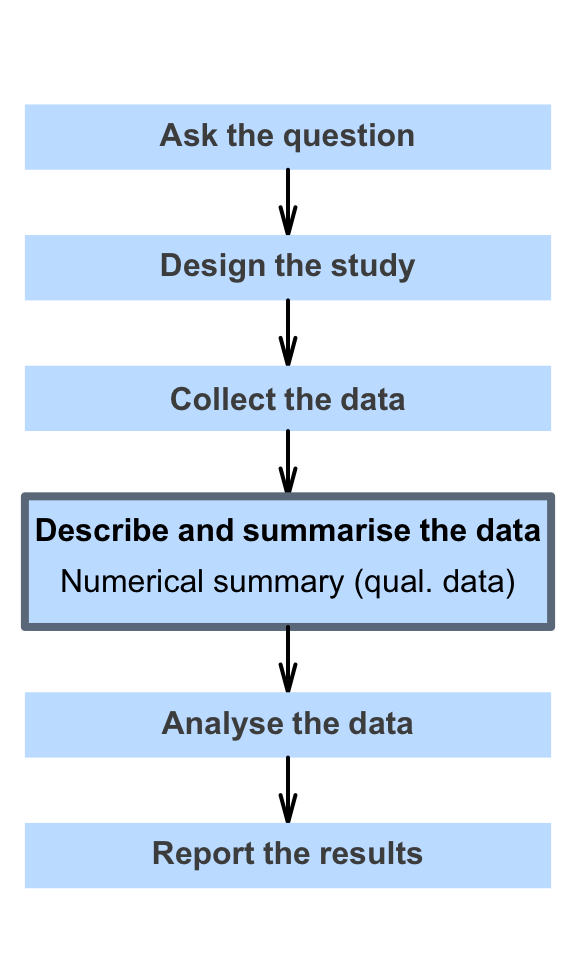# 14 Numerical summaries: qualitative data

So far, you have learnt to ask a RQ, identify different ways of obtaining data, design the study, collect the data describe the data, and graphically summarise data.

In this chapter, you will learn to numerically describe qualitative data. Both quantitative and qualitative data are described numerically in quantitative research. You will learn to:

• present and numerically summarise qualitative data.
• compute and understand row and column proportions (and percentages).
• compute and understand odds and odds ratios.
• describe relationships between qualitative variables.